## Outline

The vignette is organized as follows. In chapter 1, we guide the reader through the preparatory steps (loading packages and data). In the chapters that follow, we discuss regression estimation (with focus on weighted least squares, M- and GM-estimators) for 3 different modes of inference.

• Design-based inference (Chapter 2)
• Model-based inference (Chapter 3)
• Compound design- and model-based inference (Chapter 4)

Good to know.

Loosely speaking, the 3 modes of inference reflect how much confidence we place in the validity of our regression model. In design-based inference (also known as inference for descriptive population parameters), inference is with respect to the randomization distribution induced by the sampling—the model is of subordinate importance.

## 1 Preparations

First, we load the packages robsurvey and survey (Lumley, 2010, 2021). For regression analysis, the availability of the survey package is imperative.

IMPORTANT

It is assumed that the reader is familiar with the key functions of the survey package, like svydesign(), etc.

> library("robsurvey", quietly = TRUE)
> library("survey")

Note. The argument quietly = TRUE suppresses the start-up message in the call of library("robsurvey").

### 1.1 Data

The counties dataset contains county-specific information on population, number of farms, land area, etc. for a sample of $$n = 100$$ counties in the U.S. in the 1990s. The sampling design is simple random sample (without replacement) from the population of $$3\,141$$ counties. The dataset is tabulated in Lohr (1999, Appendix C) and is based on 1994 data of the U.S. Bureau of the Census. The first 3 rows of the data are

> data(counties)
state          county landarea totpop unemp farmpop numfarm farmacre weights
1    AL        Escambia      948  36023  1339     531     414    90646   31.41
2    AL        Marshall      567  73524  3189    1592    1582   136599   31.41
3    AK Prince of Wales     7325   6408   383      71       2      214   31.41
fpc
1 3141
2 3141
3 3141

where

 state state county county landarea land area, 1990 (square miles) totpop population total, 1992 unemp number of unemployed persons, 1991 farmpop farm population, 1990 numfarm number of farms, 1987 farmacre acreage in farms, 1987 weights sampling weight fpc finite population correction

### 1.2 Goal

The goal is to regress the county-specific size of the farm population in 1990 (variable farmpop) on a set of explanatory variables. The simplest population regression model is

$\begin{equation} \xi: \quad \mathrm{farmpop}_i = b_0 + b_1 \cdot \mathrm{numfarm}_i + \sqrt{v_i} E_i, \qquad i \in U, \end{equation}$

where $$U$$ is the set of labels of all $$3\,141$$ counties in the U.S. (population), $$b_0, b_1 \in \mathbb{R}$$ are unknown regression coefficients, $$v_i$$ are known constants (i.e., known up to a constant multiplicative factor, $$\sigma$$), and $$E_i$$ are regression errors (random variables) with mean zero and unit variance such that $$E_i$$ and $$E_j$$ are conditionally independent given $$\mathrm{numfarm}_i$$, $$i \in U$$.

Another candidate model is

$\begin{equation} \xi_{log}: \quad \log(\mathrm{farmpop}_i) = b_0 + b_1 \cdot \log(\mathrm{numfarm}_i) + \sqrt{v_i} E_i, \qquad i \in U. \end{equation}$

### 1.3 Sampling design

The counties dataset is of size $$n=100$$. In what follows, we restrict attention to the subset of the 98 counties with $$\mathrm{farmpop}_i > 0$$. Hence, we define the sampling design dn.

> dn <- svydesign(ids = ~1, fpc = ~fpc, weights = ~weights,
+     data = counties[counties$farmpop > 0, ]) ### 1.4 Exploring the data The figures below show scatterplots of farmpop plotted against numfarm (in raw and log scale). The scatterplot in the left panel indicates that the variance of farmpop increases with larger values of numfarm (heteroscedasticity). The same graph but in log-log scale (see right panel) shows an outlier, which is located far from the bulk of the data.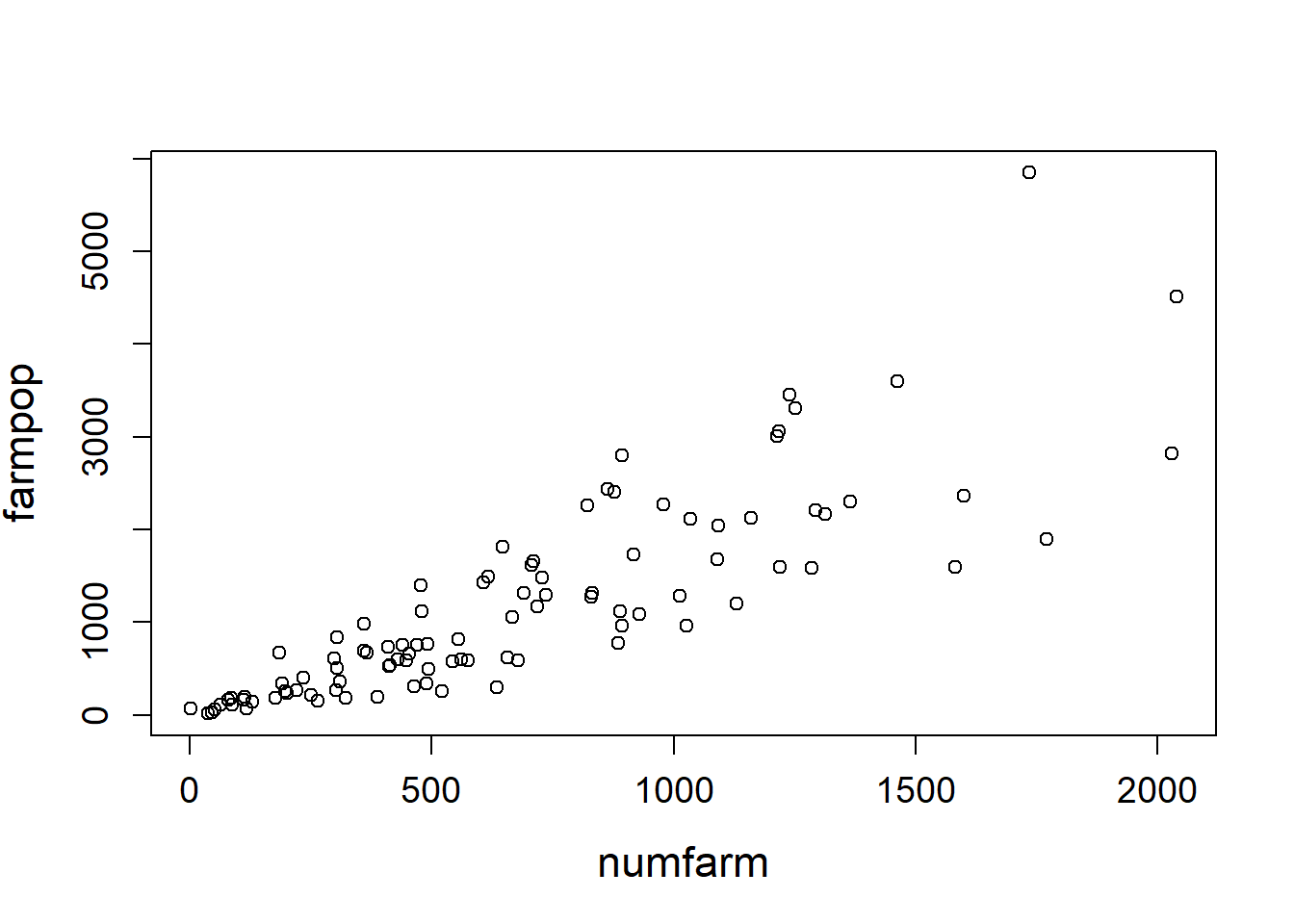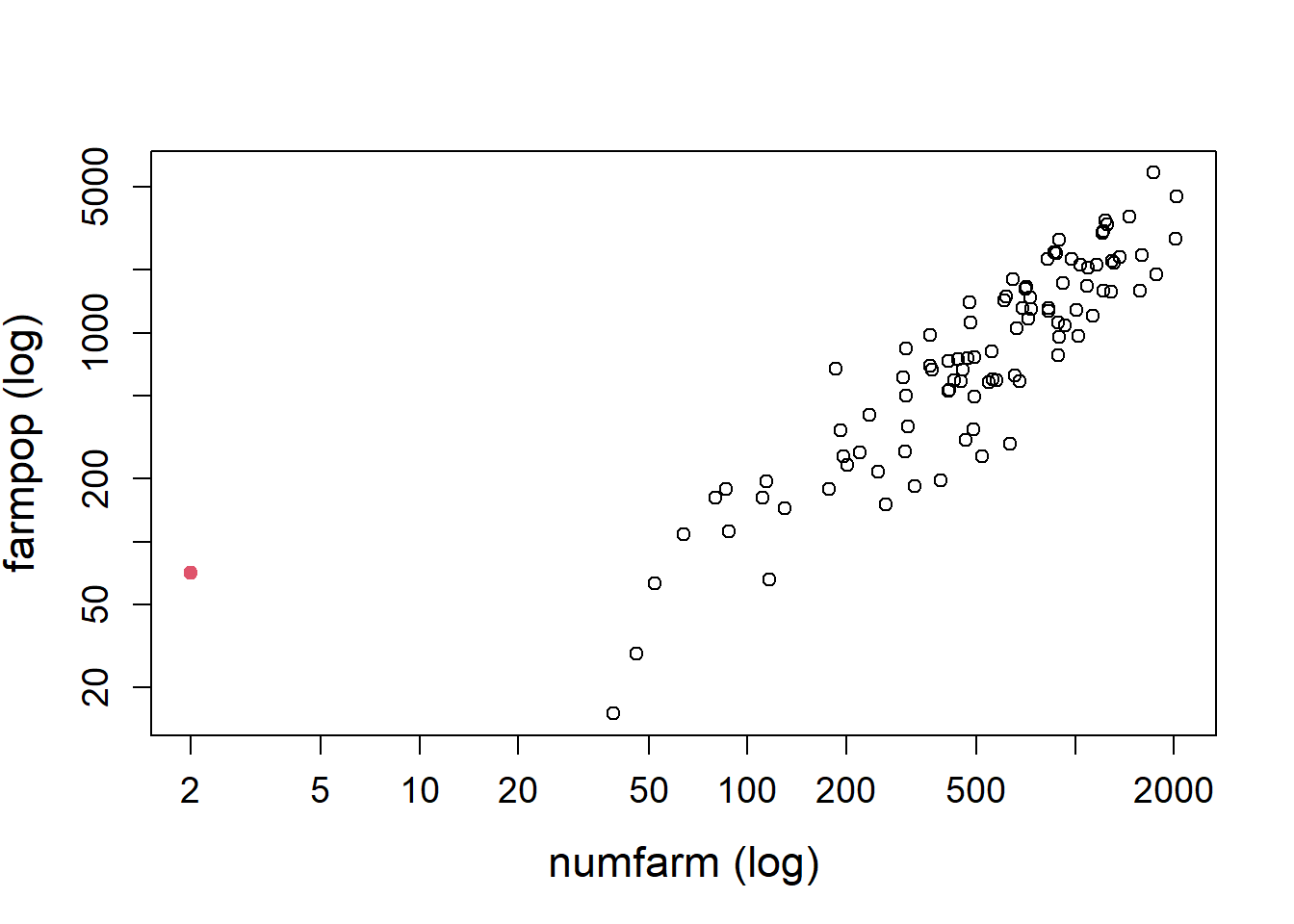Good to know. If the sampling weights were not all equal, it is helpful to use a bubble plot with circles whose area is proportional to the sampling weight; see svyplot() in the survey package. ## 2 Design-based inference We consider fitting model $$\xi$$ (under the assumption of homoscedasticity, i.e., $$v_i \equiv 1$$) by weighted least squares. The function to do so is svyreg(), and it requires two arguments: a formula object (a symbolic description of the model to be fitted) and surveydesign object (class survey::survey.design). > m <- svyreg(farmpop ~ numfarm, dn, na.rm = TRUE) > m Weighted least squares Call: svyreg(formula = farmpop ~ numfarm, design = dn, na.rm = TRUE) Coefficients: (Intercept) numfarm -121.310 1.921 Scale estimate: 563.4  The output resembles the one of an lm() call. The estimated model can be summarized using > summary(m) Call: svyreg(formula = farmpop ~ numfarm, design = dn, na.rm = TRUE) Residuals: Min. 1st Qu. Median Mean 3rd Qu. Max. -1381.71 -309.50 -13.04 0.00 181.30 2637.47 Coefficients: Estimate Std. Error t value Pr(>|t|) (Intercept) -121.3105 93.7991 -1.293 0.196 numfarm 1.9215 0.1934 9.936 <2e-16 *** --- Signif. codes: 0 '***' 0.001 '**' 0.01 '*' 0.05 '.' 0.1 ' ' 1 Residual standard error: 563.4 on 3076.18 degrees of freedom The subsequent figure shows the model diagnostic plot of the standardized residuals vs. fitted values,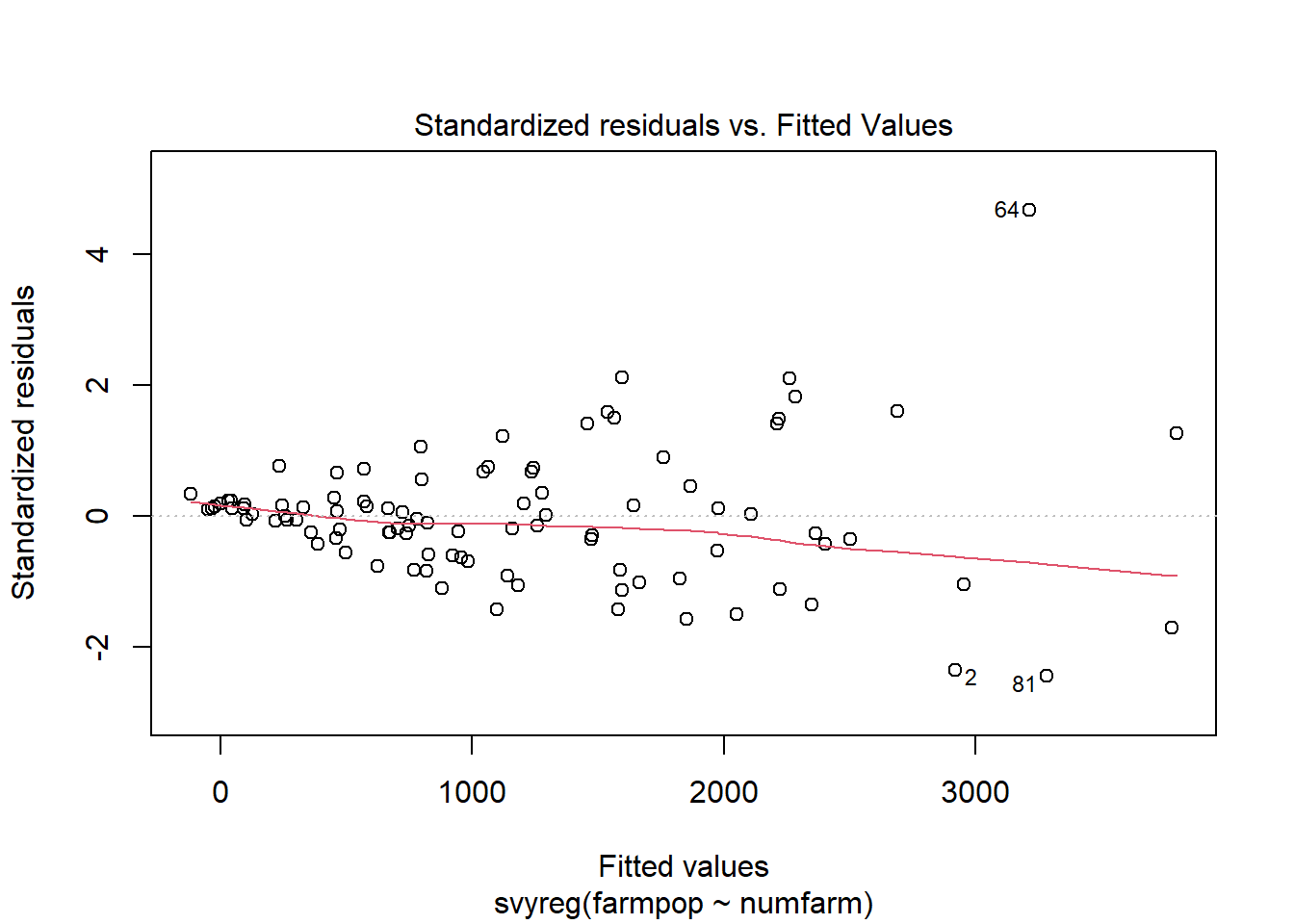Other useful methods and utility functions • coef() extracts the estimated coefficients • residuals() extracts the residuals • fitted() extracts the fitted values • vcov() extracts the variance-covariance matrix of the estimated coefficients Good to know. Alternatively, linear models can be fitted by weighted least squares with the svyglm() function in the survey package. The summary() method for survey::svyglm() computes p-values under the assumption of the Gaussian distribution, whereas our method assumes a Student t-distribution. ### 2.1 Heteroscedasticity In the above scatterplot, we observe that the variance of farmpop increases with larger values of numfarm (heteroscedasticity). We conjecture that the $$v_i$$’s in model $$\xi$$ are proportional to the square root of the variable numfarm. Thus, we add variable vi to the design object dn with the update() command > dn <- update(dn, vi = sqrt(numfarm)) The argument var of svyreg() is now used to specify the heteroscedastic variance (it can be defined as a formula, i.e. ~vi, or the variable name in quotation marks, "vi"). > svyreg(farmpop ~ -1 + numfarm, dn, var = ~vi, na.rm = TRUE) Weighted least squares Call: svyreg(formula = farmpop ~ -1 + numfarm, design = dn, var = ~vi, na.rm = TRUE) Coefficients: numfarm 1.775 Scale estimate: 99.65  Note that we have dropped the regression intercept. ### 2.2 Regression M-estimator We continue to assume the heteroscedastic model $$\xi$$, but now we compute a weighed regression M-estimator. Two types of estimators are available: • svyreg_huberM() M-estimator with Huber or asymmetric Huber $$\psi$$-function (the latter obtains by specifying argument asym = TRUE); • svyreg_tukeyM() M-estimator with Tukey biweight (bisquare) $$\psi$$-function. The tuning constant of both $$\psi$$-functions is called k. The Huber M-estimator of regression with k = 3 is (note that we use na.rm = TRUE to remove observations with missing values) > m <- svyreg_huberM(farmpop ~ -1 + numfarm, dn, var = ~vi, k = 1.3, na.rm = TRUE) > m Survey regression M-estimator (Huber psi, k = 1.3) Call: svyreg_huberM(formula = farmpop ~ -1 + numfarm, design = dn, k = 1.3, var = ~vi, na.rm = TRUE) IRWLS converged in 6 iterations Coefficients: numfarm 1.669 Scale estimate: 83.35 (weighted MAD)  and the summary obtains by > summary(m) Call: svyreg_huberM(formula = farmpop ~ -1 + numfarm, design = dn, k = 1.3, var = ~vi, na.rm = TRUE) Residuals: Min. 1st Qu. Median Mean 3rd Qu. Max. -165.951 -64.126 -10.095 6.848 47.253 458.062 Coefficients: Estimate Std. Error t value Pr(>|t|) numfarm 1.66859 0.05554 30.04 <2e-16 *** --- Signif. codes: 0 '***' 0.001 '**' 0.01 '*' 0.05 '.' 0.1 ' ' 1 Residual standard error: 83.35 on 3077.18 degrees of freedom Robustness weights: Min. 1st Qu. Median Mean 3rd Qu. Max. 0.2369 1.0000 1.0000 0.9294 1.0000 1.0000  The output of the summary method is almost identical with the one of an lm() call. The paragraph on robustness weights is a numerical summary of the M-estimator’s robustness weights, which can be extracted from the estimated model with robweights(). The subsequent figure shows the graph for plot(residuals(m), robweights(m)). From the graph, we can see by how much the residuals have been downweighted.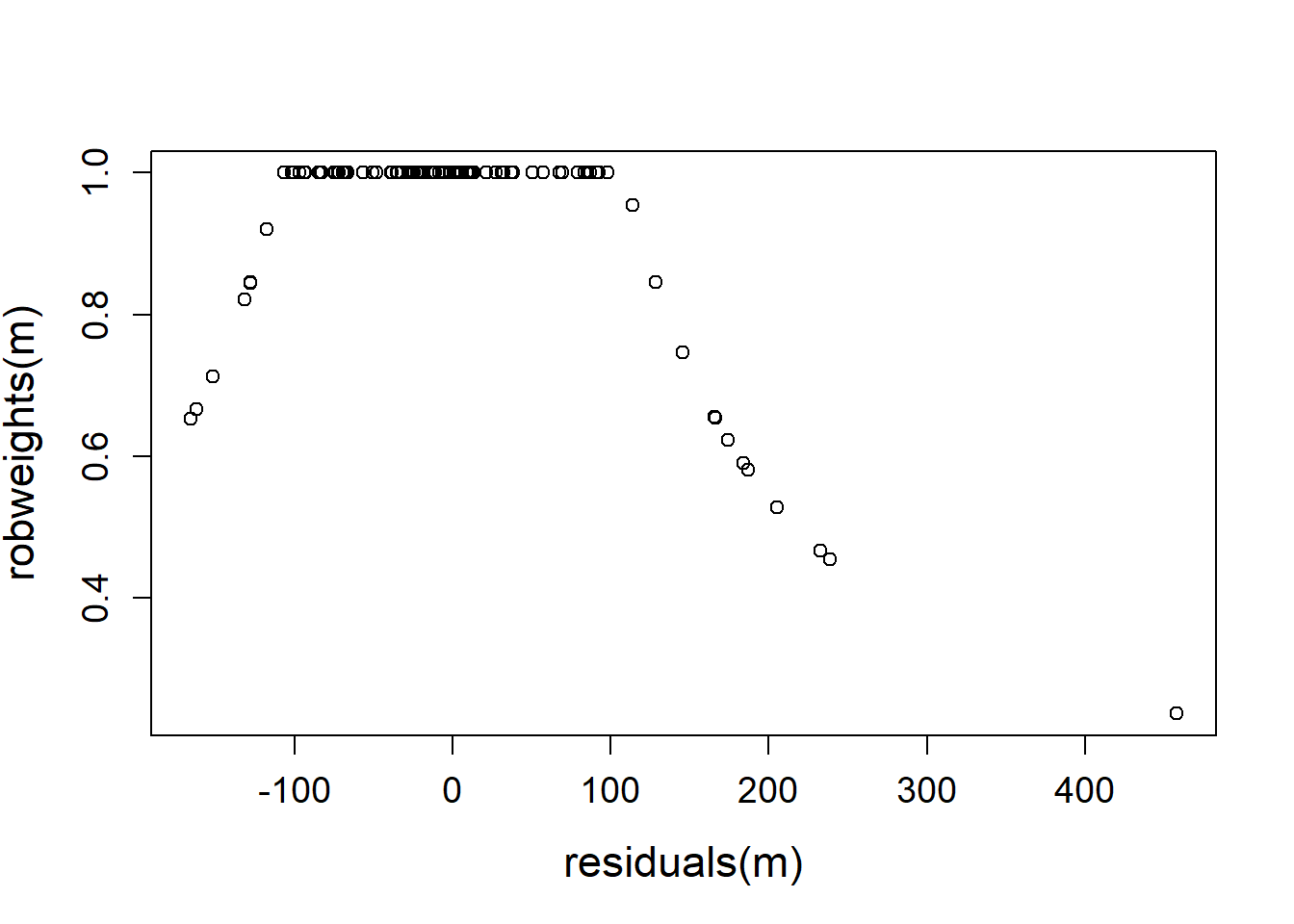IMPORTANT (failure of convergence) In the above example, the algorithm converged in 6 IRLWS (iteratively reweighted least squares) iterations. In case the algorithm does not converge, the default settings of the IRWLS algorithm can be modified. The following settings can be specified/ changed (as function arguments) in the call of svyreg_huberM() or svyreg_tukeyM(): • maxit = 50: maximum number of iterations to use • tol = 1e-5: numerical tolerance criterion to stop the iterations • init = NULL: initialization of the regression M-estimator; if init = NULL the regression estimator is initialized by weighted least squares; otherwise, init can be specified as a starting value, i.e., a $$p$$-vector of regression coefficients. See also svyreg_control(). ### 2.3 Regression GM-estimator We want to fit the population regression model $\begin{equation*} \log(\mathrm{farmpop}_i) = b_0 + b_1 \log(\mathrm{numfarm}_i) + b_2 \log(\mathrm{totpop}_i) + b_3 \log(\mathrm{farmacre}_i) + \sqrt{v_i} E_i, \qquad i \in U, \end{equation*}$ with sample data by the weighted generalized M-estimator (GM) of regression. GM-estimators are robust against high leverage observations (i.e., outliers in the design space of the model) while M-estimators of regression are not. Two types of GM-estimators are available: • svyreg_huberGM() with Huber or asymmetric Huber $$\psi$$-function (the latter obtains by specifying argument asym = TRUE); • svyreg_tukeyGM() with Tukey biweight (bisquare) $$\psi$$-function. #### Preparation Variable farmacre contains 3 missing values. For ease of analysis, we exclude the missing values from the survey.design object dn. > dn_exclude <- na.exclude(dn) The formula object of our model is > f <- log(farmpop) ~ log(numfarm) + log(totpop) + log(farmacre) With the help of the formula object f, we form a matrix of the explanatory variables (excluding the regression intercept) of our model. This matrix is called xmat. > xmat <- model.matrix(f, dn_exclude$variables)[, -1]

Below we show the pairwise scatterplots of pairs(xmat). The pairwise distributions are mostly elliptically contoured and show some outliers.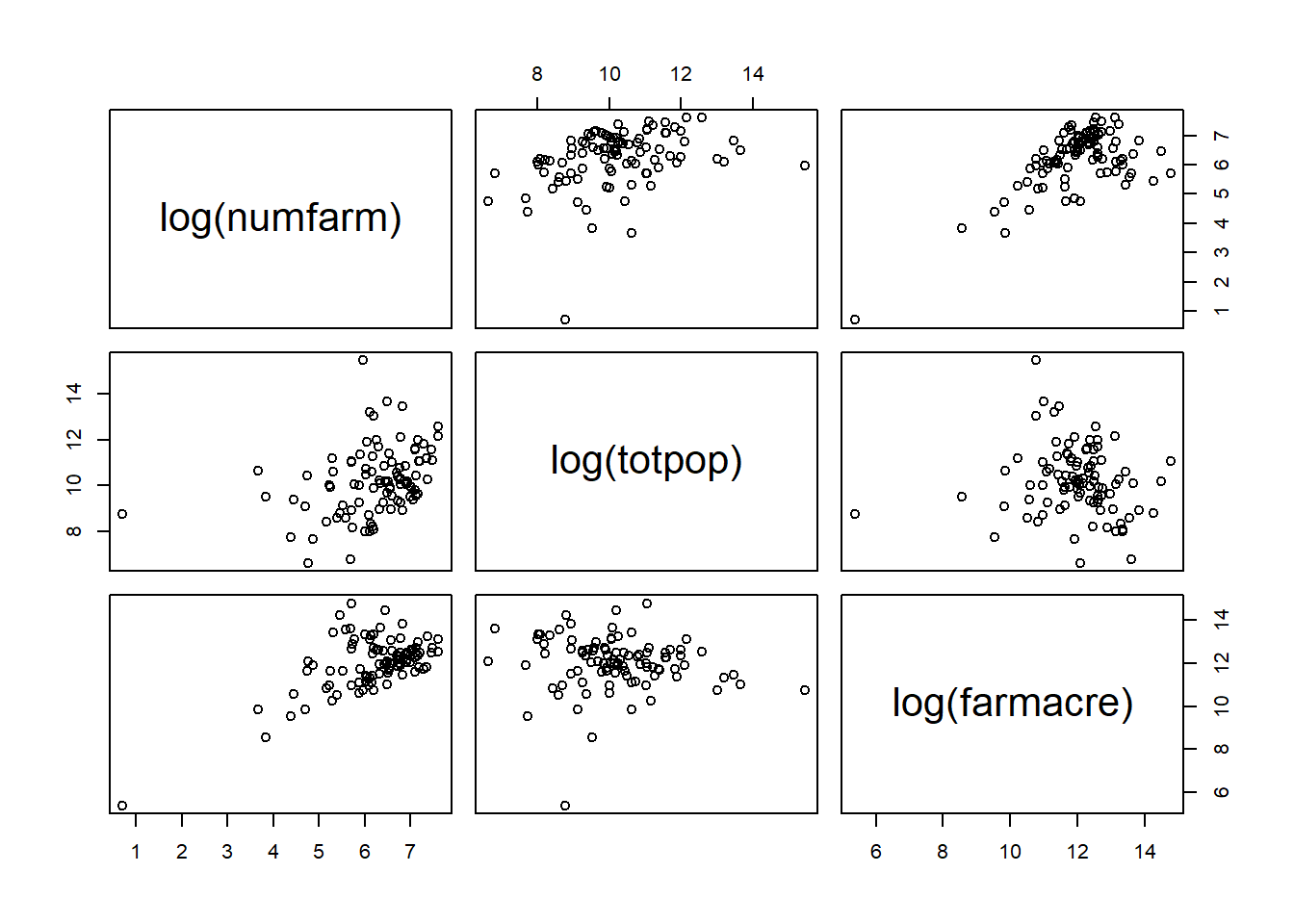#### Robust Mahalanobis distances

The intermediate goal is to compute the robust Mahalanobis distances of the observations in xmat. Observations with large distances are considered outliers and will be downweighted in the subsequent regression analysis. In order to obtain the robust Mahalanobis distances, we compute the robust multivariate location and scatter matrix of xmat using the (weighted) BACON algorithm in package wbacon (Schoch, 2021).

> library(wbacon)
> mv <- wBACON(xmat, weights = weights(dn_exclude))
> mv

Weighted BACON: Robust location, covariance, and distances
Converged in 7 iterations (alpha = 0.05)
Number of potential outliers: 2 (2.11%)

The estimated multivariate location and scatter or covariance matrix can be extracted from object mv with the functions center() and vcov(), respectively. The robust Mahalanobis distances can be extracted with

> dis <- distance(mv)

The boxplot of dis shows a couple of observations with large Mahalanobis distance. These observations are considered as potential outliers.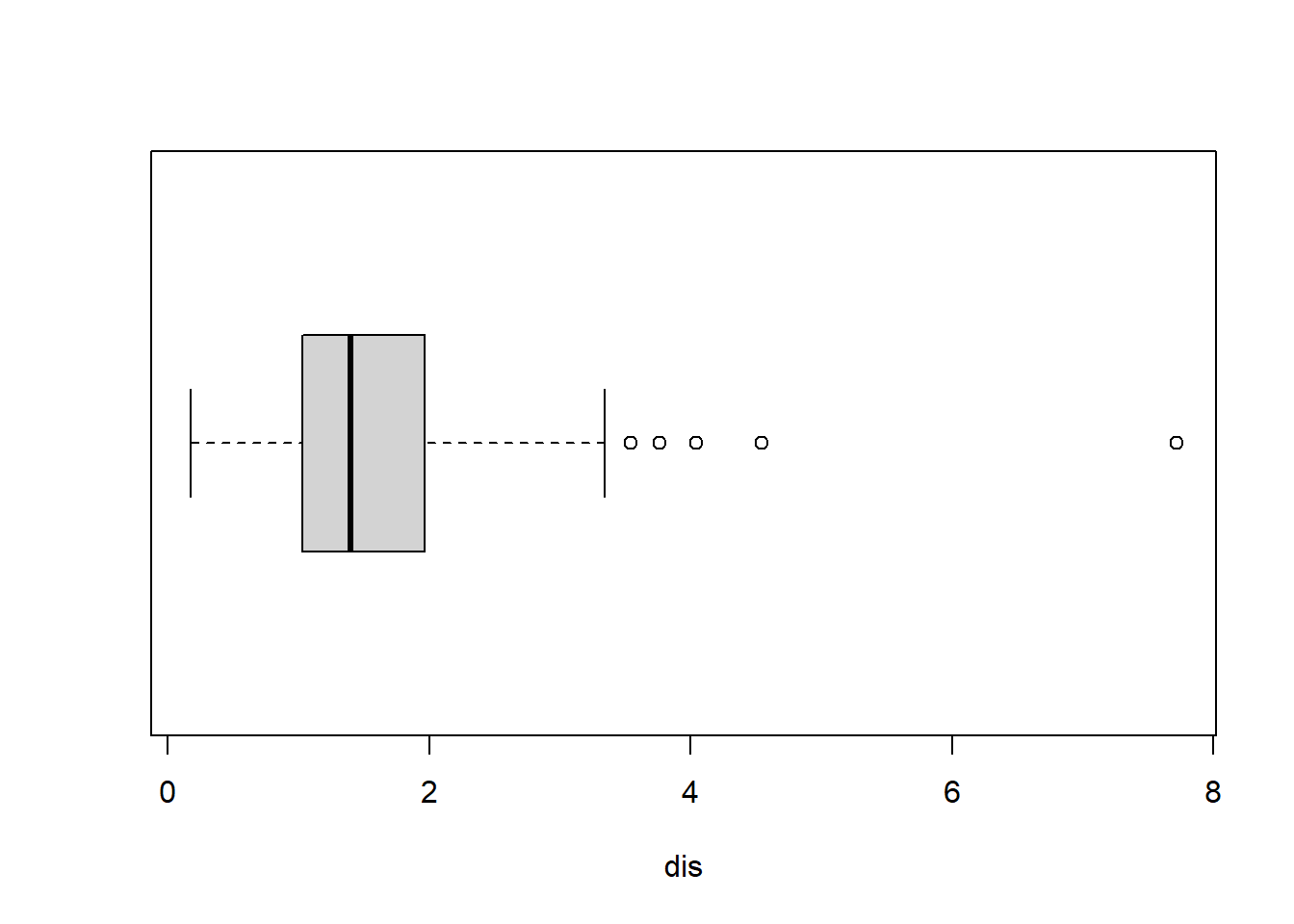Three functions are available to downweight excessively large distances (see also figure)

• tukeyWgt()
• huberWgt()
• simpsonWgt(), see Simpson et al. (1992)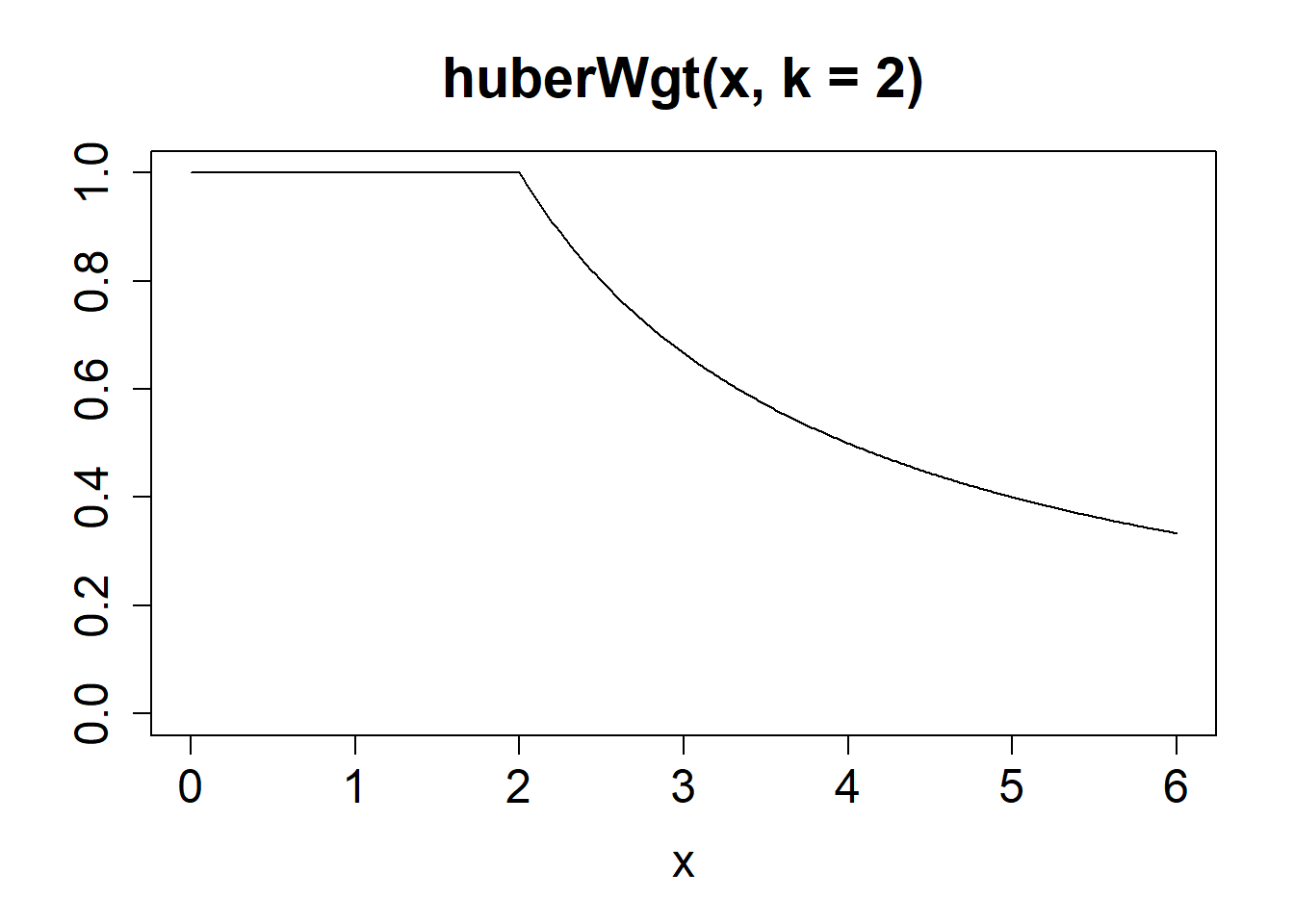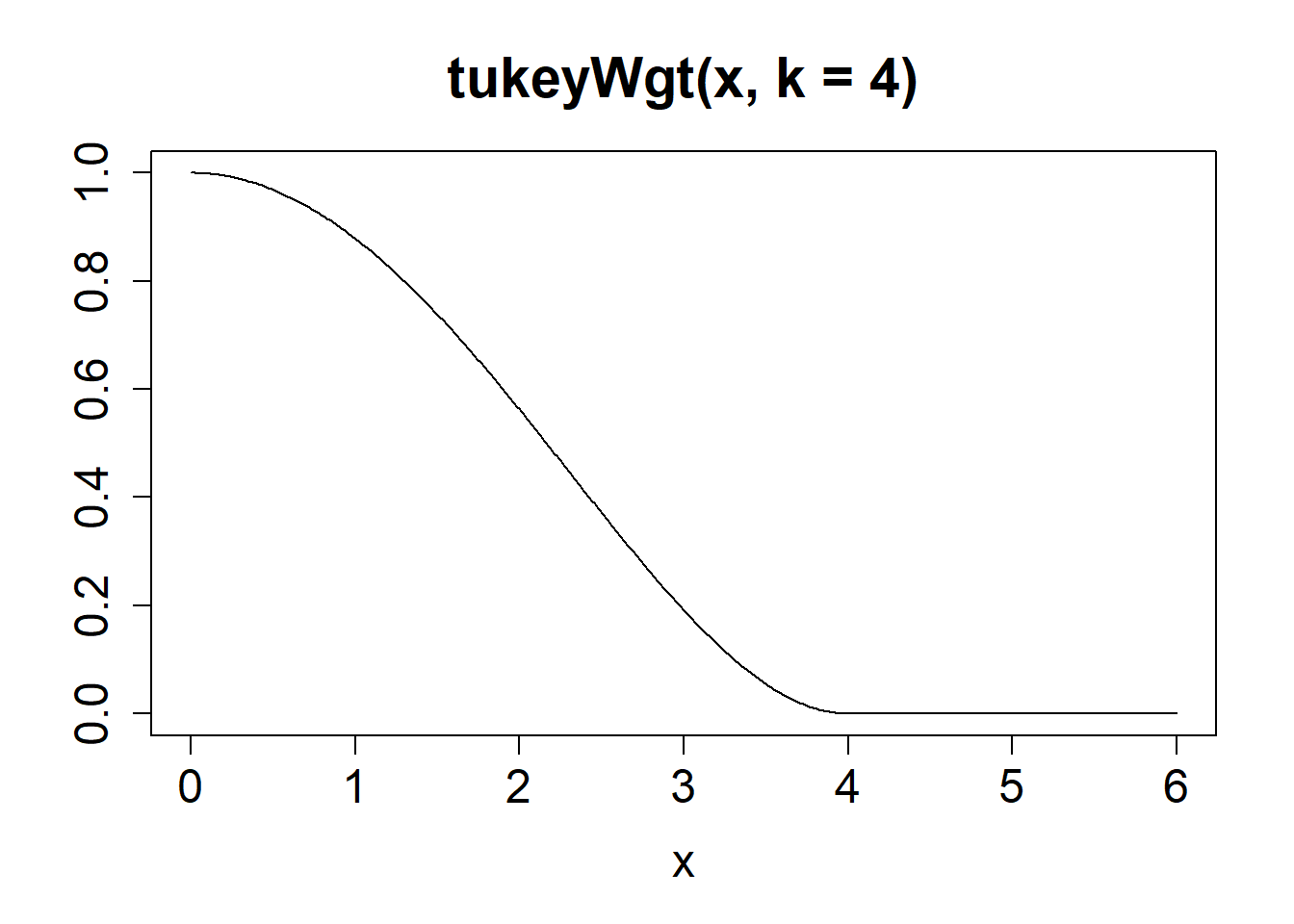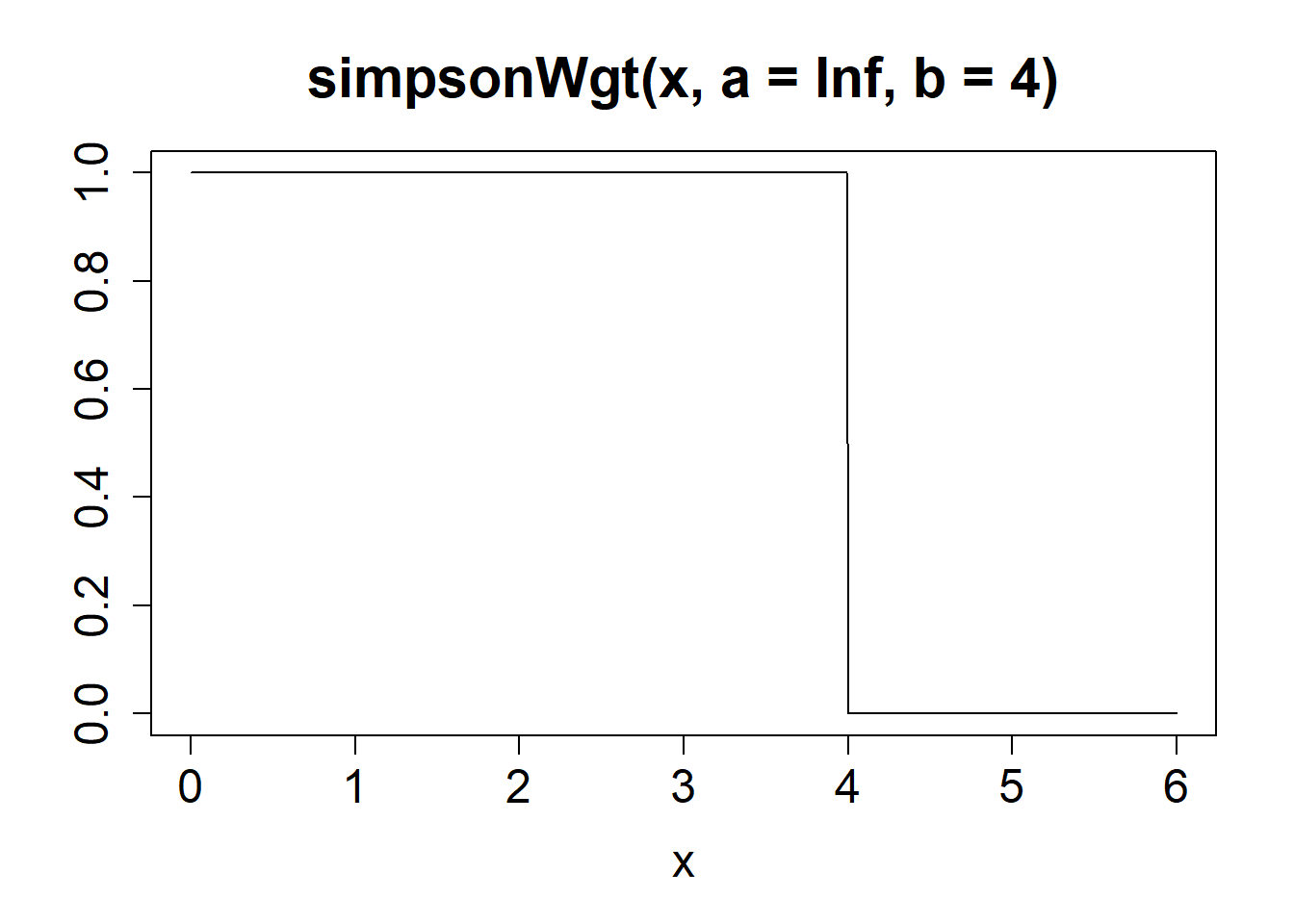#### Regression estimation and inference

The GM-estimator of regression with Tukey biweight function and downweighting of high leverage observations using tukeyWgt(dis) is

> m <- svyreg_tukeyGM(f, dn_exclude, k = 4.6, xwgt = tukeyWgt(dis))
> summary(m)

Call:
svyreg_tukeyGM(formula = f, design = dn_exclude, k = 4.6, xwgt = tukeyWgt(dis))

Residuals:
Min.   1st Qu.    Median      Mean   3rd Qu.      Max.
-1.004303 -0.352241 -0.052866  0.004878  0.360099  3.389738

Coefficients:
Estimate Std. Error t value Pr(>|t|)
(Intercept)    1.62725    0.56957   2.857  0.00431 **
log(numfarm)   1.30079    0.07044  18.466  < 2e-16 ***
log(totpop)   -0.06550    0.03176  -2.062  0.03929 *
log(farmacre) -0.20161    0.04617  -4.366 1.31e-05 ***
---
Signif. codes:  0 '***' 0.001 '**' 0.01 '*' 0.05 '.' 0.1 ' ' 1

Residual standard error: 0.5549 on 2979.95 degrees of freedom

Robustness weights:
Min. 1st Qu.  Median    Mean 3rd Qu.    Max.
0.0000  0.6270  0.7850  0.7114  0.8818  0.9959 

## 3 Model-based inference

Model-based inferential statistics are computed using a dummy survey.design object under the assumption of a simple random sample without replacement. We define

> dn0 <- svydesign(ids = ~1, weights = ~1,
+     data = counties[counties$farmpop > 0, ]) which differs from our original design dn in the following aspects: • weights = ~1 (i.e., the sampling weights are set to 1.0); • the argument fpc (finite sampling correction) is not specified. We consider fitting model $$\xi_{log}$$ by the Huber regression M-estimator (based on the design object dn0) > m <- svyreg_huberM(log(farmpop) ~ log(numfarm), dn0, k = 1.3, na.rm = TRUE) In the call of summary(), we specify the argument mode = "model" for model-based inference (default is mode = "design") to obtain > summary(m, mode = "model") Call: svyreg_huberM(formula = log(farmpop) ~ log(numfarm), design = dn0, k = 1.3, na.rm = TRUE) Residuals: Min. 1st Qu. Median Mean 3rd Qu. Max. -1.203816 -0.314133 0.007801 0.003245 0.335280 3.647648 Coefficients: Estimate Std. Error t value Pr(>|t|) (Intercept) -0.13991 0.29129 -0.48 0.632 log(numfarm) 1.08916 0.04663 23.36 <2e-16 *** --- Signif. codes: 0 '***' 0.001 '**' 0.01 '*' 0.05 '.' 0.1 ' ' 1 Residual standard error: 0.4934 on 96 degrees of freedom Robustness weights: Min. 1st Qu. Median Mean 3rd Qu. Max. 0.1758 1.0000 1.0000 0.9557 1.0000 1.0000  Likewise, we can call vcov(m, mode = "model") to extract the estimated model-based covariance matrix of the regression estimator. Good to know (comparison with function rlm in package MASS). For the purpose of comparison, we have fitted the above model with the function rlm in package MASS (Venables and Ripley, 2002). > library(MASS) > summary(rlm(log(farmpop) ~ log(numfarm), + data = counties[counties$farmpop > 0, ],
+     k = 1.3, na.action = na.omit))

Call: rlm(formula = log(farmpop) ~ log(numfarm), data = counties[counties\$farmpop >
0, ], k = 1.3, na.action = na.omit)
Residuals:
Min        1Q    Median        3Q       Max
-1.203817 -0.314142  0.007801  0.335269  3.647595

Coefficients:
Value   Std. Error t value
(Intercept)  -0.1399  0.2913    -0.4801
log(numfarm)  1.0892  0.0466    23.3564

Residual standard error: 0.4934 on 96 degrees of freedom

## 4 Compound design- and model-based inference

Suppose we wish to estimate the regression coefficients that refer to the superpopulation (i.e., a more general population than the finite population). Furthermore, our sample data represent a large fraction of the finite population (i.e., $$n/N$$ is not small). In statistical inference about the coefficients, we need to account for the sampling design and the model because the quantity of interest is the superpopulation parameter and the sampling fraction is not negligible (Rubin-Bleuer and Schiopu-Kratina, 2005; Binder and Roberts, 2009); see also motivating example.

Motivating example

Let $$\bar{y}$$ be the mean of a sample of size $$n$$. For ease of discussion, we shall assume a simple random sample (without replacement) from a finite population of size $$N$$. The mean of the finite population is denoted by $$\bar{Y}$$. Here, we wish to refer to a more general population than the finite population. Therefore, $$\bar{y}$$ is regarded as an estimator of the super-population mean $$\mu$$ with the following decomposition

$\sqrt{n} (\bar{y} - \mu) = \sqrt{n}(\overline{y} - \overline{Y}) + \sqrt{\frac{n}{N}} \cdot \sqrt{N}(\overline{Y} - \mu).$

If the sampling fraction, $$n/N$$, is small, the second term on the right-hand side is negligible regarding the limiting distribution of $$\sqrt{n}(\bar{y} - \mu)$$—therefore design-based inference is only concerned with $$\sqrt{n}(\bar{y} - \bar{Y})$$. If, however, the sampling fraction is not small, statistical inference about $$\mu$$ should take the limiting distribution of $$\sqrt{N}(\bar{Y} - \mu)$$ into account.

### 4.1 The MU284 population

The MU284 population of Särndal et al. (1992, Appendix B) is a dataset with observations on the 284 municipalities in Sweden in the late 1970s and early 1980s. It is available in the sampling package; see Tillé and Matei (2021). The population is divided into two parts based on 1975 population size (P75):

• the MU281 population, which consists of the 281 smallest municipalities;
• the MU3 population of the three biggest municipalities/ cities in Sweden (Stockholm, Göteborg, and Malmö).

The three biggest cities take exceedingly large values (representative outliers) on almost all of the variables. To account for this (at least to some extent), a stratified sample has been drawn from the MU284 population using a take-all stratum. The sample data, MU284strat, is of size $$n=60$$ and consists of

• a stratified simple random sample (without replacement) from the MU281 population, where stratification is by geographic region (REG) with proportional sample size allocation;
• a take-all stratum that includes the three biggest cities/ municipalities (population M3).
 Stratum $$S_1$$ $$S_2$$ $$S_3$$ $$S_4$$ $$S_5$$ $$S_6$$ $$S_7$$ $$S_8$$ take all Population stratum size 24 48 32 37 55 41 15 29 3 Sample stratum size 5 10 6 8 11 8 3 6 3

The overall sampling fraction is $$60/284 \approx 21\%$$ (and thus not negligible). The data frame MU284strat includes the following variables.

 LABEL identifier variable P85 1985 population size (in $$10^3$$) P75 1975 population size (in $$10^3$$) RMT85 revenues from the 1985 municipal taxation (in $$10^6$$ kronor) CS82 number of Conservative seats in municipal council SS82 number of Social-Democrat seats in municipal council (1982) S82 total number of seats in municipal council in 1982 ME84 number of municipal employees in 1984 REV84 real estate values in 1984 (in $$10^6$$ kronor) CL cluster indicator REG geographic region indicator Stratum stratum indicator weights sampling weights fpc finite population correction

We load the data and generate the sampling design.

> data(MU284strat)
> dn <- svydesign(ids = ~1, strata = ~ Stratum, fpc = ~fpc, weights = ~weights,
+     data = MU284strat)

### 4.2 Model fit and statistical inference

The Huber M-estimator of regression is

> m <- svyreg_huberM(RMT85 ~ REV84 + P85 + S82 + CS82, dn, k = 2)

The compound design- and model-based distribution of the estimated coefficients obtains by specifying the argument mode = "compound" in the call of the summary() method.

> summary(m, mode = "compound")

Call:
svyreg_huberM(formula = RMT85 ~ REV84 + P85 + S82 + CS82, design = dn,
k = 2)

Residuals:
Min.  1st Qu.   Median     Mean  3rd Qu.     Max.
-77.877  -18.469    5.044   66.250   17.635 2690.755

Coefficients:
Estimate Std. Error t value Pr(>|t|)
(Intercept) 130.973688  22.260303   5.884 1.15e-08 ***
REV84         0.005729   0.003304   1.734   0.0840 .
P85           9.499801   0.285577  33.265  < 2e-16 ***
S82          -3.845513   0.542752  -7.085 1.14e-11 ***
CS82         -1.965082   1.010420  -1.945   0.0528 .
---
Signif. codes:  0 '***' 0.001 '**' 0.01 '*' 0.05 '.' 0.1 ' ' 1

Residual standard error: 23.96 on 279 degrees of freedom

Robustness weights:
Min. 1st Qu.  Median    Mean 3rd Qu.    Max.
0.01781 1.00000 1.00000 0.95076 1.00000 1.00000 

We may call vcov(m, mode = "compound") to extract the estimated covariance matrix of the regression estimator under the compound design- and model-based distribution.

## References

BINDER, D. A. AND ROBERTS, G. (2009). Design- and Model-Based Inference for Model Parameters. In: Sample Surveys: Inference and Analysis ed. by Pfeffermann, D. and Rao, C. R. Volume 29B of Handbook of Statistics, Amsterdam: Elsevier, Chap. 24, 33–54. DOI: 10.1016/ S0169-7161(09)00224-7

LOHR, S. L. (1999). Sampling: Design and Analysis, Pacific Grove (CA): Duxbury Press.

LUMLEY, T. (2010). Complex Surveys: A Guide to Analysis Using R: A Guide to Analysis Using R, Hoboken (NJ): John Wiley and Sons.

LUMLEY, T. (2021). survey: analysis of complex survey samples. R package version 4.0, URL https://CRAN.R-project.org/package=survey.

RUBIN-BLEUER, S. AND SCHIOPU-KRATINA, I. (2005). On the Two-phase framework for joint model and design-based inference. The Annals of Statistics 33, 2789–2810. DOI: 10.1214/ 009053605000000651

SÄRNDAL, C.-E., SWENSSON, B. AND WRETMAN, J. (1992). Model Assisted Survey Sampling, New York: Springer.

SCHOCH, T. (2021). wbacon: Weighted BACON algorithms for multivariate outlier nomination (detection) and robust linear regression. Journal of Open Source Software 6, 3238. DOI: 10. 21105/joss.03238

SIMPSON, D. G., RUPPERT, D. AND CARROLL, R. J. (1992). On one-step GM estimates and stability of inferences in linear regression. Journal of the American Statistical Association 87, 439–450. DOI: 10.2307/2290275

TILLE, T. and MATEI, A. (2021). sampling: Survey Sampling. R package version 2.9. https://CRAN.R-project.org/package=sampling.

VENABLES, W. N. AND RIPLEY, B. D. (2002). Modern Applied Statistics with S, New York: Springer, 4th edition. DOI: 10.1007/978-0-387-21706-2